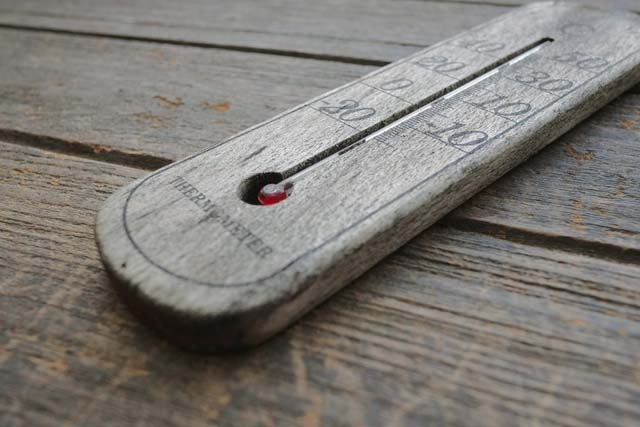## \$hide=homeKelvin and Fahrenheit are two important temperature scales. Kelvin is a standard metric scale, with a degree the same size as the Celsius degree, but with its zero point at absolute zero. Fahrenheit is the temperature, most commonly used in the United States. Fortunately, it's simple to convert between the two scales, providing you know the equation.

### Kelvin To Fahrenheit Conversion Formula

Here is the formula to convert Kelvin to Fahrenheit:
° F = 9/5(K - 273) + 32
or you may see the equation using more significant figures as:
° F = 9/5(K - 273.15) + 32
or
° F = 1.8(K - 273) + 32

You can use whichever equation you prefer.

It is easy to convert Kelvin to Fahrenheit with these four steps.
1. Subtract 273.15 from your Kelvin temperature

2. Multiply this number by 1.8 (this is the decimal value of 9/5).

3. Add 32 to this number.
Kelvin To Fahrenheit Conversion Example
Let's try a sample problem, converting room temperature in Kelvin to degrees Fahrenheit.
Room temperature is 293K.
Start with the equation (I chose the one with fewer significant figures):
° F = 9/5(K - 273) + 32
Plug in the value for Kelvin:
F = 9/5(293 - 273) + 32
Doing the math:
F = 9/5(20) + 32
F = 36 + 32
F = 68
Fahrenheit is expressed using degrees, so the answer is that room temperature is 68°F.

### Fahrenheit To Kelvin Conversion Example

Let's try the conversion the other way. For example, say you want to convert human body temperature, 98.6°F, into its Kelvin equivalent. You can use the same equation:

F = 9/5(K - 273) + 32
98.6 = 9/5(K - 273) + 32
Subtract 32 from both sides to get:
66.6 = 9/5(K - 273)

Multiple 9/5 times the values inside the parenthesis to get:
66.6 = 9/5K - 491.4

Get the variable (K) on one side of the equation. I chose to subtract (-491.4) from both sides of the equation, which is the same as adding 491.4 to 66.6:
558 = 9/5K

Multiply both sides of the equation by 5 to get:
2790 = 9K

Finally, divide both sides of the equation by 9 to get the answer in K:
310 = K

So, human body temperature in Kelvin is 310 K. Remember, Kelvin temperature is not expressed using degrees, just a capital letter K.

Note: You could have used another form of the equation, simply rewritten to solve for the Fahrenheit to Kelvin conversion:
K = 5/9(F - 32) + 273.15

which is basically the same as saying Kelvin equals the Celsius value plus 273.15.## |Featured Content_\$type=three\$c=3\$l=0\$m=0\$s=hide\$rm=0

Name

Advice,95,Balance Work & Life,62,Be a Better Manager,33,Break Room,12,Business Skills,119,Career,86,Career Advice,299,Career Care,1,Career Choice,145,Career Growth,332,Career Paths,24,Career Problem,8,Education,121,Entrepreneurship,46,Featured,78,Features,468,Finance,14,Freelancing,1,Internship,11,Interview Tips,45,Job Search,48,Leadership,84,Marketing,10,Money & Career,21,Resume,12,Tools & Skills,19,Training,2,Work Environment,111,Work From Home,10,
ltr
item
Career Magazine: How to Convert Kelvin to Fahrenheit
How to Convert Kelvin to Fahrenheit
https://1.bp.blogspot.com/-0tDtKOzWf9g/V02aovRkylI/AAAAAAAAWuQ/tCLzmgkrcJQaG913h4poQLSkUHQXC_v7wCLcB/s1600/dear.jpg
https://1.bp.blogspot.com/-0tDtKOzWf9g/V02aovRkylI/AAAAAAAAWuQ/tCLzmgkrcJQaG913h4poQLSkUHQXC_v7wCLcB/s72-c/dear.jpg
Career Magazine
https://career.dearjulius.com/2016/05/how-to-convert-kelvin-to-fahrenheit.html
https://career.dearjulius.com/
https://career.dearjulius.com/
https://career.dearjulius.com/2016/05/how-to-convert-kelvin-to-fahrenheit.html
true
709333744733904694
UTF-8